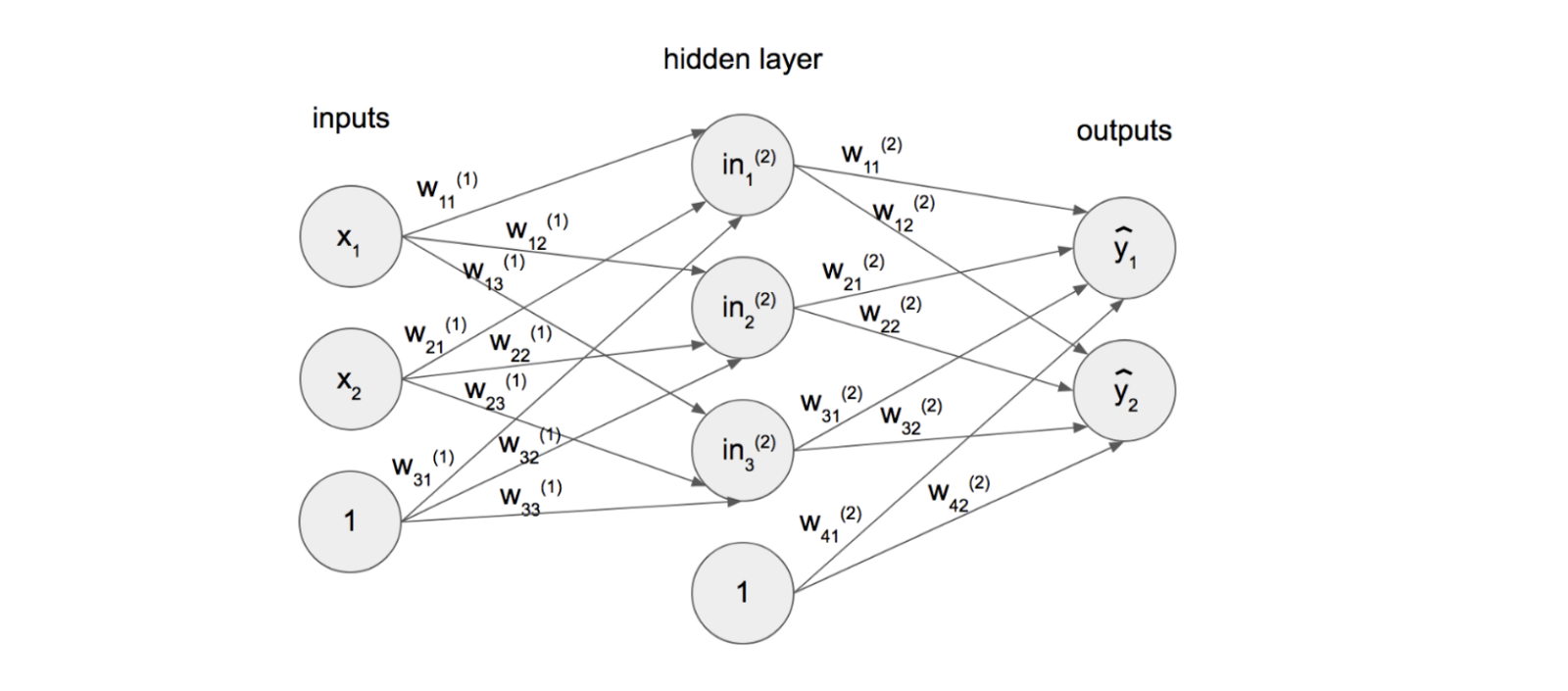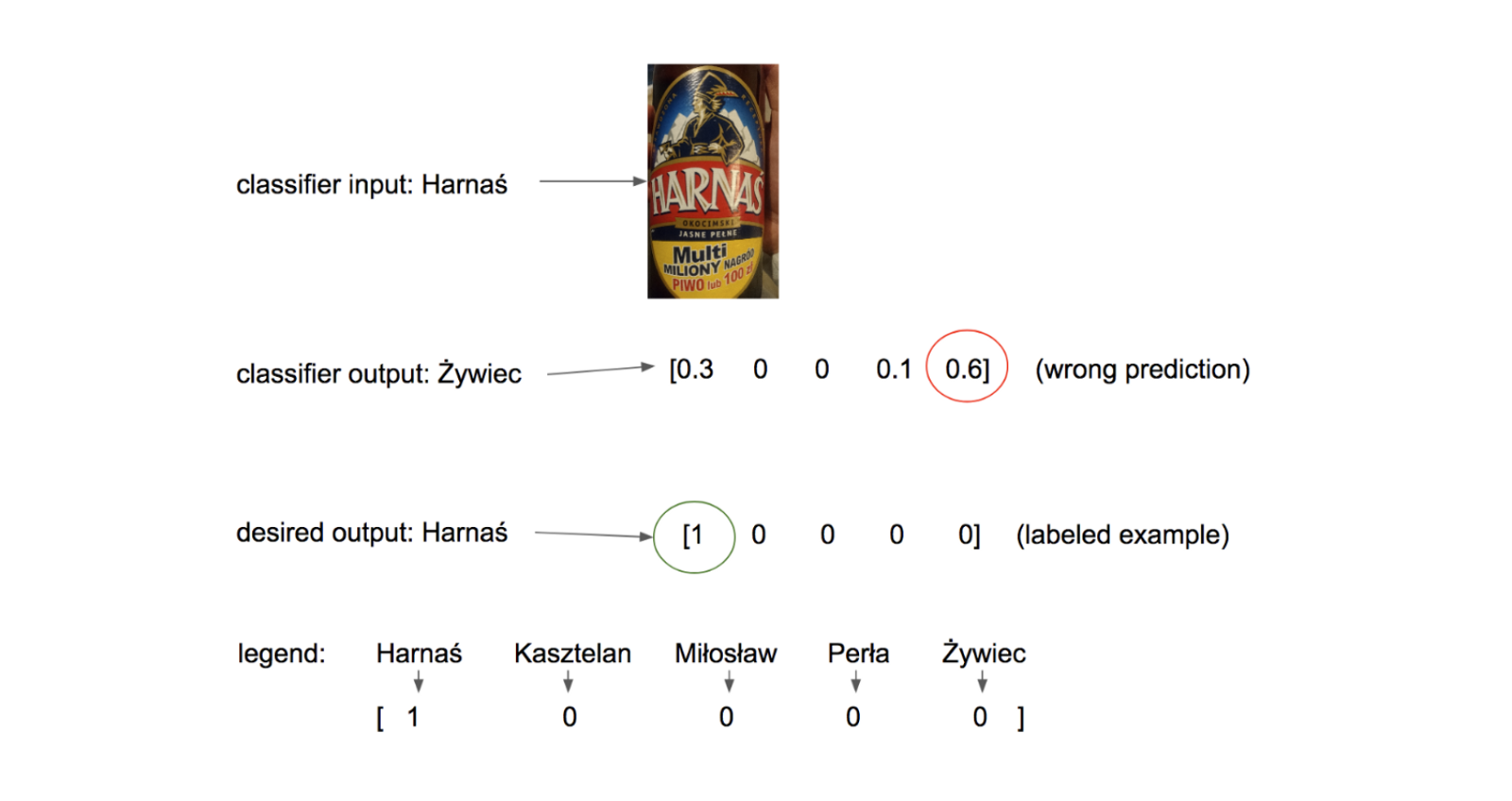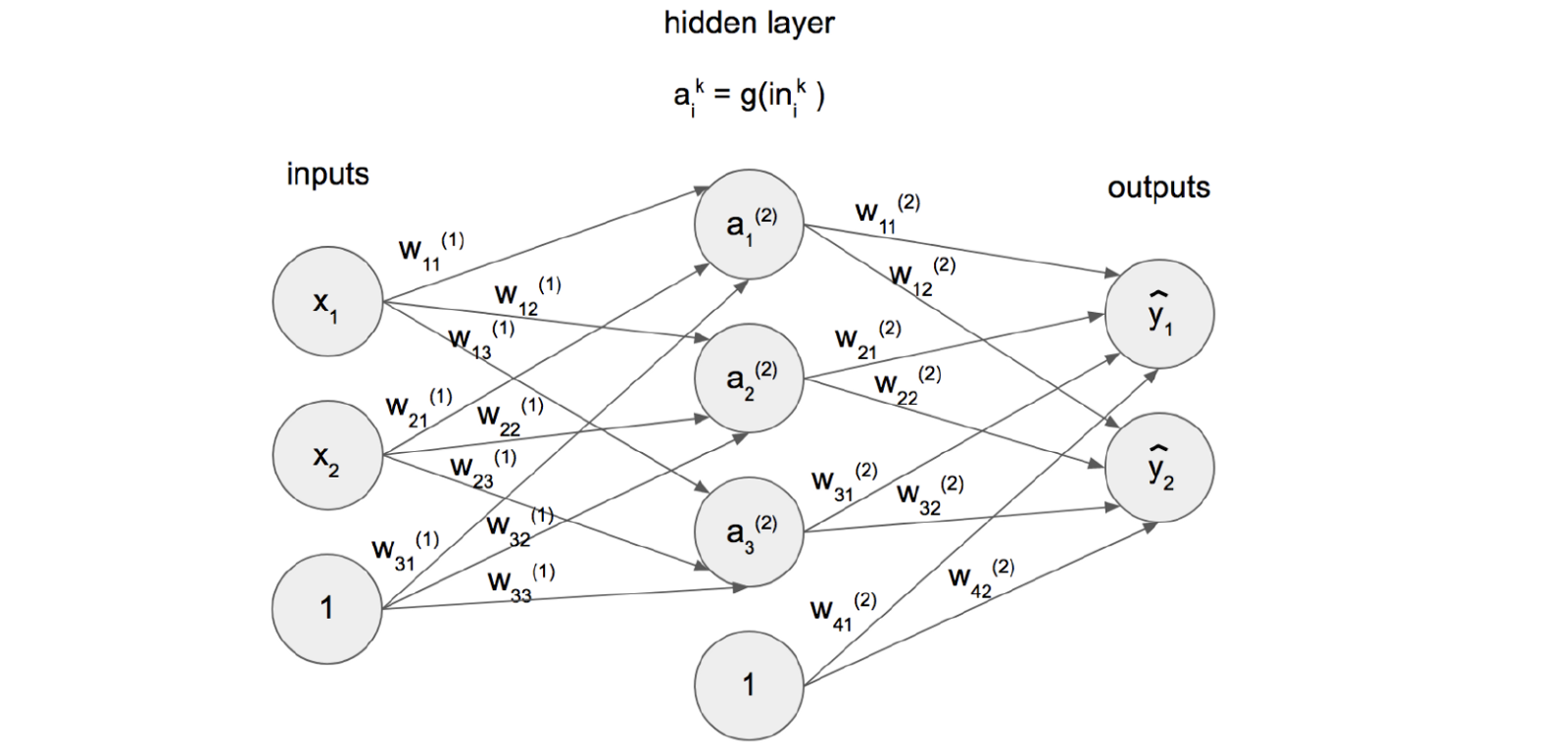in

# Let me introduce you to neural networksThis article provides an intuitive approach to neural networks and their learning process (backpropagation) using some simple undergraduate mathematical formulas and terms. Being a huge believer in value of knowledge sharing, I hope it’ll shed some light for someone starting AI/ML journey or clarify few things for those mindlessly using high-level tools such as Keras (been there, done that). Without further ado…

It’s reasonable to think of a neural network (NN) as a mathematical function, which in practice tends to be very complicated because of three things:
1. 1) it has a large number of coefficients (weights), often exceeding tens of millions,
2) it’s a very deeply nested function, hence even simple gradient calculation (partial derivative) is relatively slower,
3) most of its computations are performed on multidimensional tensors.

Figure 1 contains a popular representation of a simple neural network with three basic building blocks: unit (single circle with value `in` , input `x`, output `y` or bias `1`), layer (units arranged into one vertical group) and weight (connection between units with value `w` representing its strength). Equation 1, 2, 3, 4 and 5 translate this graphical representation to mathematical formula.Figure 1: A simple neural network with one hidden layer (a layer between inputs and outputs).

“Perceptron” is a common name for a neural network, where inputs are immediately coupled with outputs (no hidden layers, unlike in Figure 1). The presence of hidden (middle) layer of units, which prevents from direct connections between inputs and outputs, allows neural network to model highly nonlinear mathematical functions. Norvig and Russell justify that, using XOR gate as an example, in a following manner: “[…] linear classifiers […] can represent linear decision boundaries in the input space. This works fine for the carry function, which is a logical AND […]. The sum function, however, is an XOR (exclusive OR) of the two inputs. […] this function is not linearly separable so the perceptron cannot learn it. The linearly separable functions constitute just a small fraction of all Boolean functions.” (P. Norvig and S. J. Russell, Artificial Intelligence: A Modern Approach, Prentice Hall,
2010).

Before delving into learning process of NNs, it’s important to make two additions to previous model:
1) error function (also called cost function),
2) activation function.

Ad 1. The most reliable way for the algorithm to represent predictions is through a vector of probabilities. Consider an example of beer name predictions based on image of label. Figure 2 shows a probability output of a classifier (notice that all values sum to 1), compared with an output, that it should strive for. A cost function, introduced in this section, called categorical cross entropy (Equation 6), simply measures the correlation between those two probability distributions (predicted and ideal). Notice that multiplication by one-hot encoded examples, forces the function to only compare non-zero elements of ideal distribution, with respective values of classifier output further from 1 being penalized more than values close to 1 (thanks to the nature of logarithm).Figure 2: Exemplary classifier input, output and desired (one-hot encoded) output with legend.where:d_i is the ith element of one-hot encoded (desired) probability vector d,p_i is the ith element of probability vector p predicted by the classifier.

Ad 2. Unit’s value `in` is rarely propagated explicitly to next layers. So called activation function is used instead. The one introduced in this section is called sigmoid (Equation 7). The updated model of simple neural network from Figure 1 is shown in Figure 3. One thing worth pointing out is a difference between sigmoid and softmax function (Equation 8) — both used in artificial neural networks. Whereas sigmoid inputs a single value and outputs a normalized scalar, softmax inputs a list of values and outputs a vector of real numbers in range [0, 1] that add up to 1, thus can be interpreted as a probability distribution. Sigmoid is used in hidden units, while softmax is usually applied in the last output layer. Both functions can be categorized as logistic functions.Figure 3: Updated model of simple neural network from Figure 1. g is a sigmoid activation function. Outputs are often normalized using softmax.Softmax function s(z)_j “squashes” a K-dimensional vector z to K-dimensional probability distribution,where j = 1, …, K.

The goal of neural network’s learning process is to find correct weights, i. e. weights, that will result in a mathematical model, where the difference of inputs is clearly represented in the difference of output vectors, which are subjects to analysis and prediction. For example in a trained dog breed classifier, the output vector for an image of german shepherd is clearly different than for york’s. This can be easily interpreted and lead to correct human-readable prediction of a breed. Currently, the best known way to train a network is via algorithm called backpropagation. Main idea of this method is to calculate gradients of a cost function E (e. g. categorical cross entropy) with respect to each of weights, which are later updated by some portion of these gradients as illustrated in Equation 9.

Let us consider a neural network in Figure 4 with three units, one hidden layer and sigmoid activation function. Before conducting backpropagation, so called, forward pass was performed, which simply is a mathematical inference of outputs, given inputs (Equation 10).Figure 4: A simple neural network, with sigmoid activation function g (Equation 7).

As mentioned previously, NN’s learning algorithm is based on calculating partial derivatives with respect to each of weights. A deep nesting of functions, representing more complicated networks, encourages to make use of chain rule. Figure 5 outlines a single step of backpropagation using categorical cross entropy error function E. Equation 11 and Equation 12 present symbolic gradient calculations, necessary for learning process to occur. At this point, a beautifully simple derivative of sigmoid function is worth recalling:Figure 5: Outline of a single step of backpropagation with chain rule.

With symbolic computations behind, consider following inputs to neural network from Figure 5:1) A training example. NN should strive for returning 1, whenever the input is equal to 10.2) Randomly initialized weights for first forward pass (Equation 10).

Primary step of learning process is performing inference, given randomly initialized weights and input. The produced outcome is:

which is quite far from desired 1. Backward pass allows to calculate gradients with respect to each weight, namely:

After applying update rule from Equation 9, with learning rate alpha = 0.5, new weights are:

and produce outcome:

Much closer to desired 1! Presented algorithm is iterative. With increased amount of repetitions of above step and larger amount of examples, it should converge to optimal weights (globally or locally).

Originally published at towardsdatascience.com on January 4, 2018.Android Developer

5